Скачать презентацию Transport Theory Introduction Phase space picture Beam ellipse

d65831f45a56d6636334ff7cd74e17c4.ppt

• Количество слайдов: 35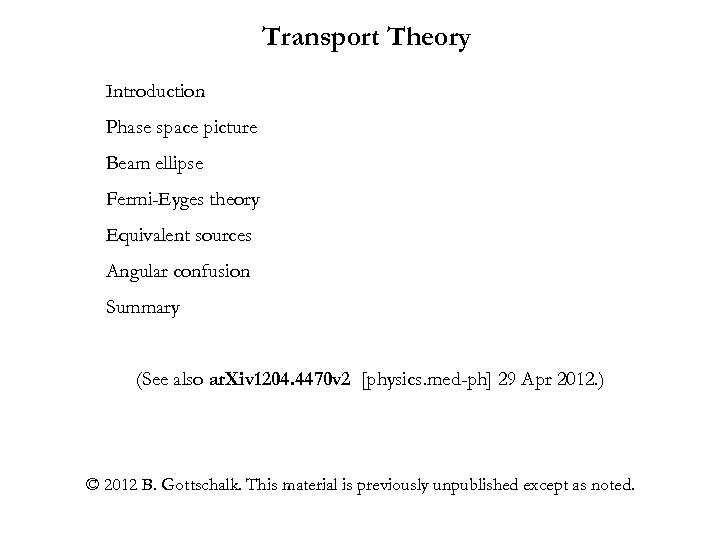Transport Theory Introduction Phase space picture Beam ellipse Fermi-Eyges theory Equivalent sources Angular confusion Summary (See also ar. Xiv 1204. 4470 v 2 [physics. med-ph] 29 Apr 2012. ) © 2012 B. Gottschalk. This material is previously unpublished except as noted.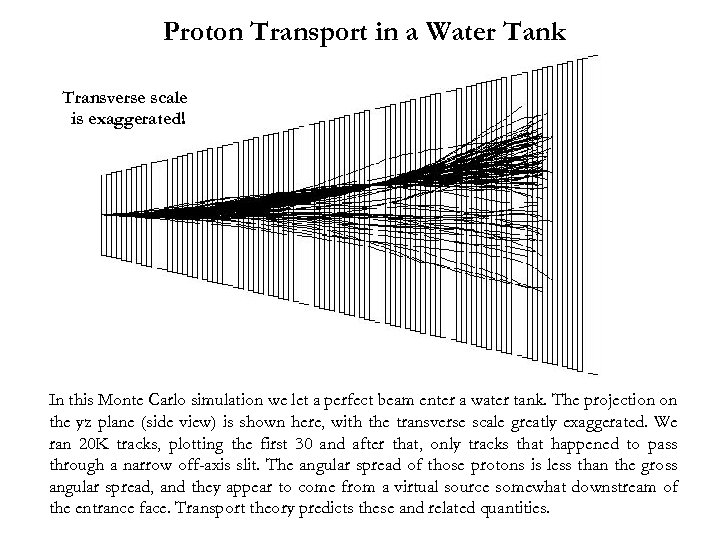Proton Transport in a Water Tank Transverse scale is exaggerated! In this Monte Carlo simulation we let a perfect beam enter a water tank. The projection on the yz plane (side view) is shown here, with the transverse scale greatly exaggerated. We ran 20 K tracks, plotting the first 30 and after that, only tracks that happened to pass through a narrow off-axis slit. The angular spread of those protons is less than the gross angular spread, and they appear to come from a virtual source somewhat downstream of the entrance face. Transport theory predicts these and related quantities.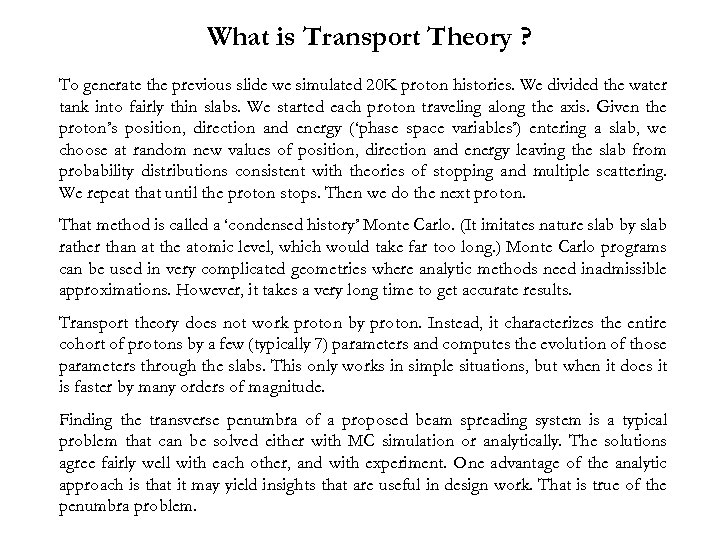What is Transport Theory ? To generate the previous slide we simulated 20 K proton histories. We divided the water tank into fairly thin slabs. We started each proton traveling along the axis. Given the proton’s position, direction and energy (‘phase space variables’) entering a slab, we choose at random new values of position, direction and energy leaving the slab from probability distributions consistent with theories of stopping and multiple scattering. We repeat that until the proton stops. Then we do the next proton. That method is called a ‘condensed history’ Monte Carlo. (It imitates nature slab by slab rather than at the atomic level, which would take far too long. ) Monte Carlo programs can be used in very complicated geometries where analytic methods need inadmissible approximations. However, it takes a very long time to get accurate results. Transport theory does not work proton by proton. Instead, it characterizes the entire cohort of protons by a few (typically 7) parameters and computes the evolution of those parameters through the slabs. This only works in simple situations, but when it does it is faster by many orders of magnitude. Finding the transverse penumbra of a proposed beam spreading system is a typical problem that can be solved either with MC simulation or analytically. The solutions agree fairly well with each other, and with experiment. One advantage of the analytic approach is that it may yield insights that are useful in design work. That is true of the penumbra problem.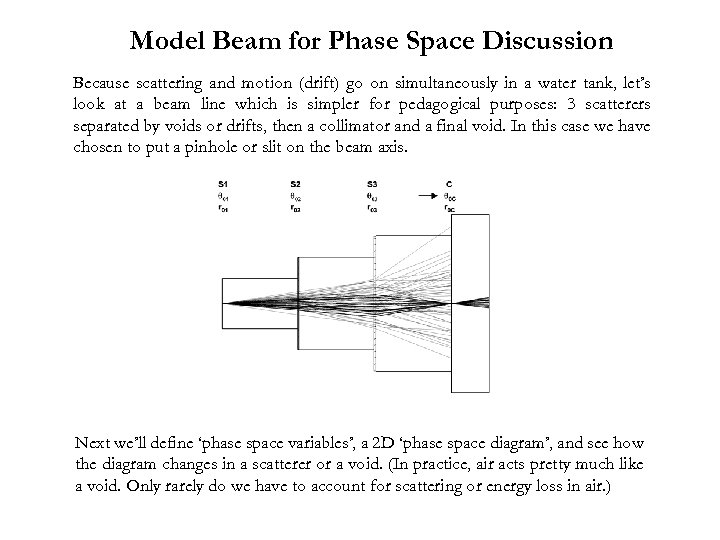Model Beam for Phase Space Discussion Because scattering and motion (drift) go on simultaneously in a water tank, let’s look at a beam line which is simpler for pedagogical purposes: 3 scatterers separated by voids or drifts, then a collimator and a final void. In this case we have chosen to put a pinhole or slit on the beam axis. Next we’ll define ‘phase space variables’, a 2 D ‘phase space diagram’, and see how the diagram changes in a scatterer or a void. (In practice, air acts pretty much like a void. Only rarely do we have to account for scattering or energy loss in air. )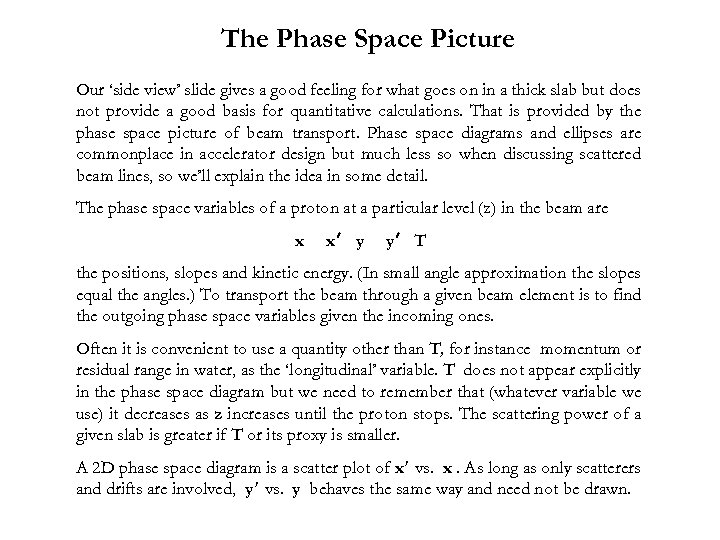The Phase Space Picture Our ‘side view’ slide gives a good feeling for what goes on in a thick slab but does not provide a good basis for quantitative calculations. That is provided by the phase space picture of beam transport. Phase space diagrams and ellipses are commonplace in accelerator design but much less so when discussing scattered beam lines, so we’ll explain the idea in some detail. The phase space variables of a proton at a particular level (z) in the beam are x x′ y y′ T the positions, slopes and kinetic energy. (In small angle approximation the slopes equal the angles. ) To transport the beam through a given beam element is to find the outgoing phase space variables given the incoming ones. Often it is convenient to use a quantity other than T, for instance momentum or residual range in water, as the ‘longitudinal’ variable. T does not appear explicitly in the phase space diagram but we need to remember that (whatever variable we use) it decreases as z increases until the proton stops. The scattering power of a given slab is greater if T or its proxy is smaller. A 2 D phase space diagram is a scatter plot of x′ vs. x. As long as only scatterers and drifts are involved, y′ vs. y behaves the same way and need not be drawn.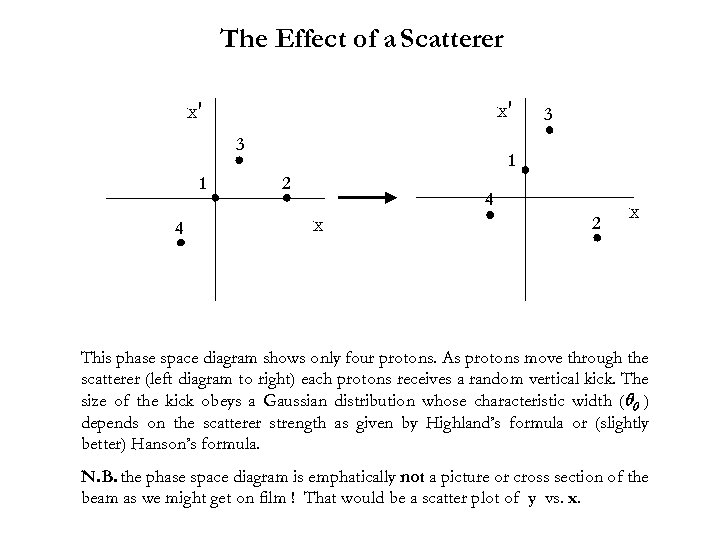The Effect of a Scatterer x′ x′ 3 1 4 3 1 2 4 x 2 x This phase space diagram shows only four protons. As protons move through the scatterer (left diagram to right) each protons receives a random vertical kick. The size of the kick obeys a Gaussian distribution whose characteristic width (θ 0 ) depends on the scatterer strength as given by Highland’s formula or (slightly better) Hanson’s formula. N. B. the phase space diagram is emphatically not a picture or cross section of the beam as we might get on film ! That would be a scatter plot of y vs. x.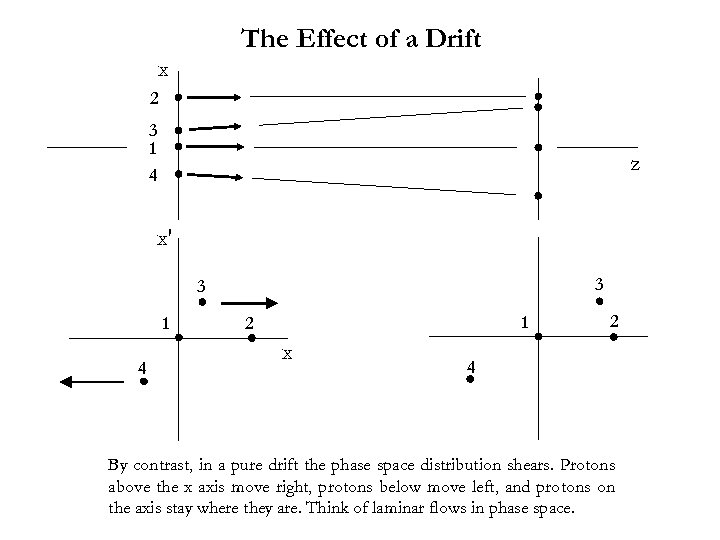The Effect of a Drift x 2 3 1 4 z x′ 3 3 1 4 1 2 x 2 4 By contrast, in a pure drift the phase space distribution shears. Protons above the x axis move right, protons below move left, and protons on the axis stay where they are. Think of laminar flows in phase space.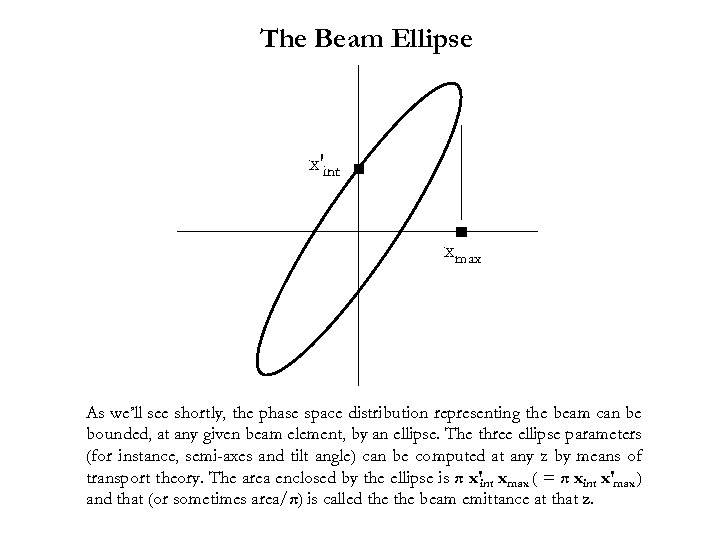The Beam Ellipse x′int xmax As we’ll see shortly, the phase space distribution representing the beam can be bounded, at any given beam element, by an ellipse. The three ellipse parameters (for instance, semi-axes and tilt angle) can be computed at any z by means of transport theory. The area enclosed by the ellipse is π x′int xmax ( = π xint x′max ) and that (or sometimes area/π) is called the beam emittance at that z.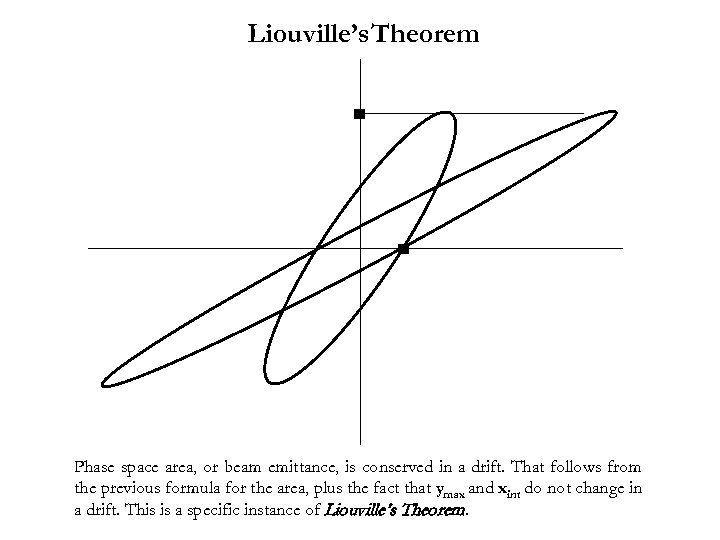Liouville’s Theorem Phase space area, or beam emittance, is conserved in a drift. That follows from the previous formula for the area, plus the fact that ymax and xint do not change in a drift. This is a specific instance of Liouville’s Theorem.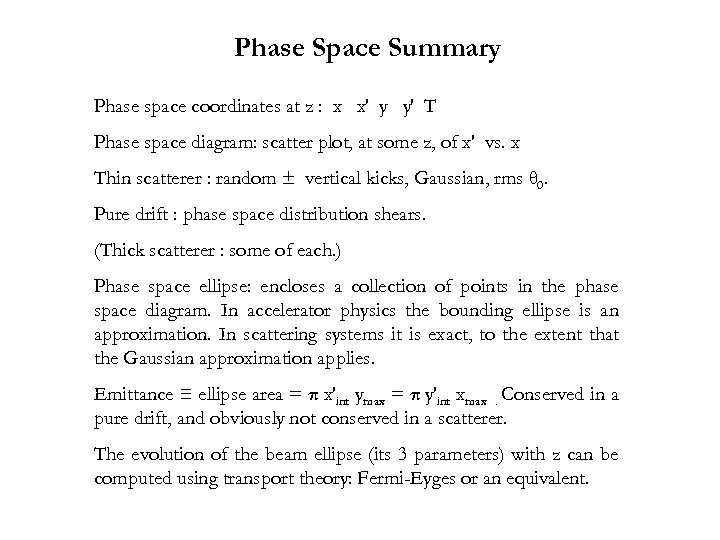Phase Space Summary Phase space coordinates at z : x x′ y y′ T Phase space diagram: scatter plot, at some z, of x′ vs. x Thin scatterer : random ± vertical kicks, Gaussian, rms θ 0. Pure drift : phase space distribution shears. (Thick scatterer : some of each. ) Phase space ellipse: encloses a collection of points in the phase space diagram. In accelerator physics the bounding ellipse is an approximation. In scattering systems it is exact, to the extent that the Gaussian approximation applies. Emittance ≡ ellipse area = π x′int ymax = π y′int xmax. Conserved in a pure drift, and obviously not conserved in a scatterer. The evolution of the beam ellipse (its 3 parameters) with z can be computed using transport theory: Fermi-Eyges or an equivalent.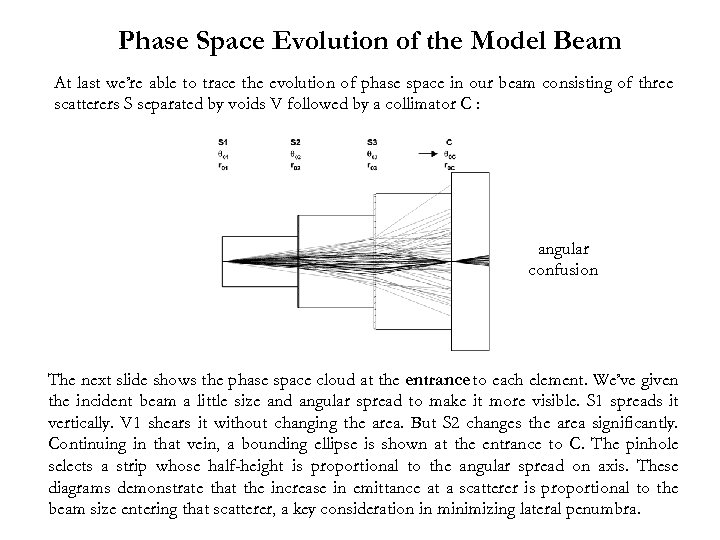Phase Space Evolution of the Model Beam At last we’re able to trace the evolution of phase space in our beam consisting of three scatterers S separated by voids V followed by a collimator C : angular confusion The next slide shows the phase space cloud at the entrance to each element. We’ve given the incident beam a little size and angular spread to make it more visible. S 1 spreads it vertically. V 1 shears it without changing the area. But S 2 changes the area significantly. Continuing in that vein, a bounding ellipse is shown at the entrance to C. The pinhole selects a strip whose half-height is proportional to the angular spread on axis. These diagrams demonstrate that the increase in emittance at a scatterer is proportional to the beam size entering that scatterer, a key consideration in minimizing lateral penumbra.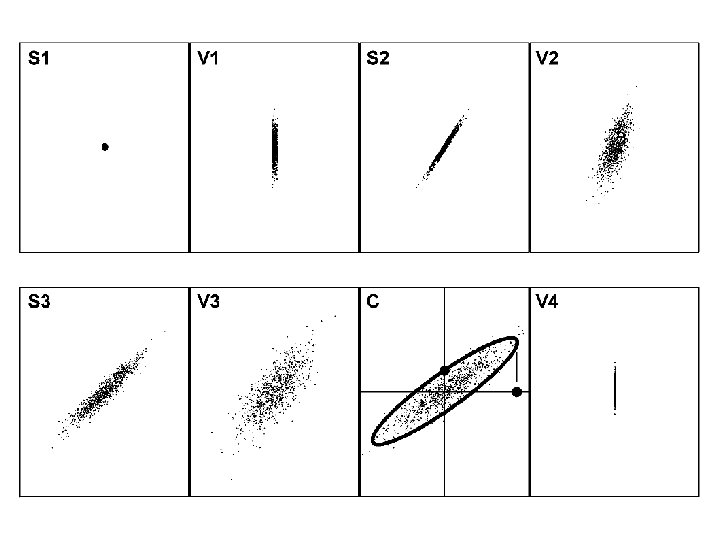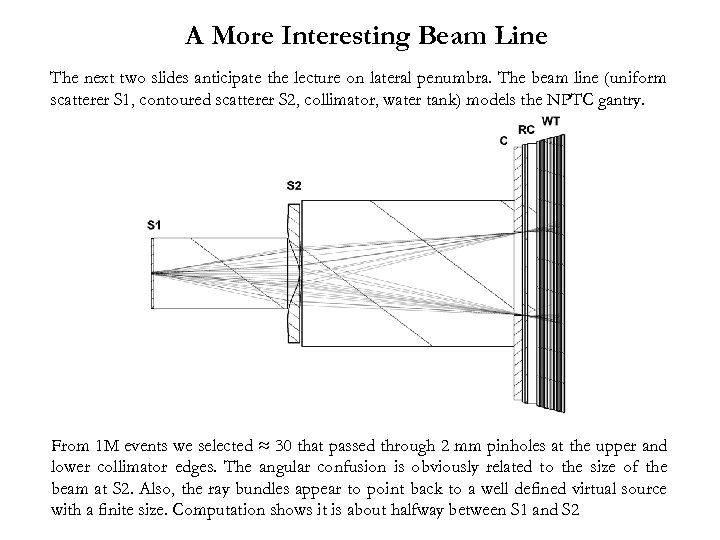A More Interesting Beam Line The next two slides anticipate the lecture on lateral penumbra. The beam line (uniform scatterer S 1, contoured scatterer S 2, collimator, water tank) models the NPTC gantry. From 1 M events we selected ≈ 30 that passed through 2 mm pinholes at the upper and lower collimator edges. The angular confusion is obviously related to the size of the beam at S 2. Also, the ray bundles appear to point back to a well defined virtual source with a finite size. Computation shows it is about halfway between S 1 and S 2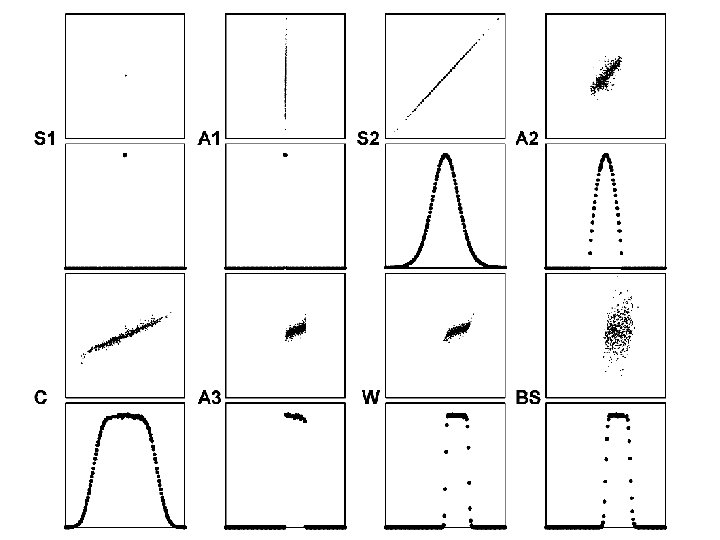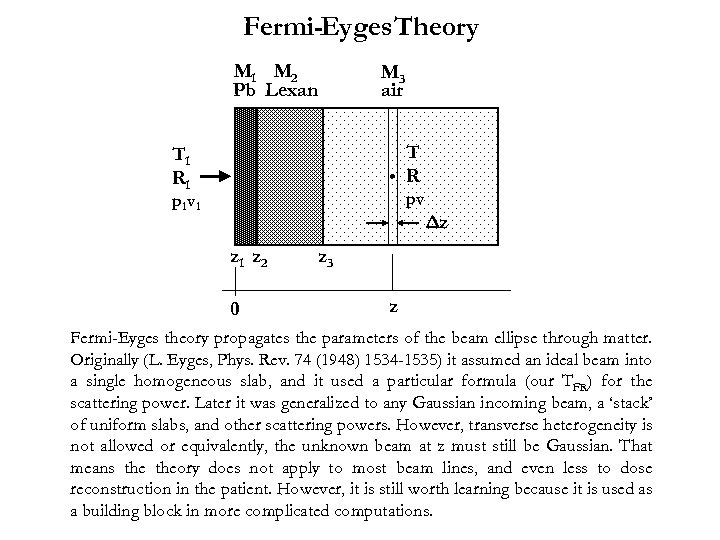Fermi-Eyges Theory M 1 M 2 Pb Lexan M 3 air T R T 1 R 1 pv p 1 v 1 Δz z 1 z 2 0 z 3 z Fermi-Eyges theory propagates the parameters of the beam ellipse through matter. Originally (L. Eyges, Phys. Rev. 74 (1948) 1534 -1535) it assumed an ideal beam into a single homogeneous slab, and it used a particular formula (our TFR) for the scattering power. Later it was generalized to any Gaussian incoming beam, a ‘stack’ of uniform slabs, and other scattering powers. However, transverse heterogeneity is not allowed or equivalently, the unknown beam at z must still be Gaussian. That means theory does not apply to most beam lines, and even less to dose reconstruction in the patient. However, it is still worth learning because it is used as a building block in more complicated computations.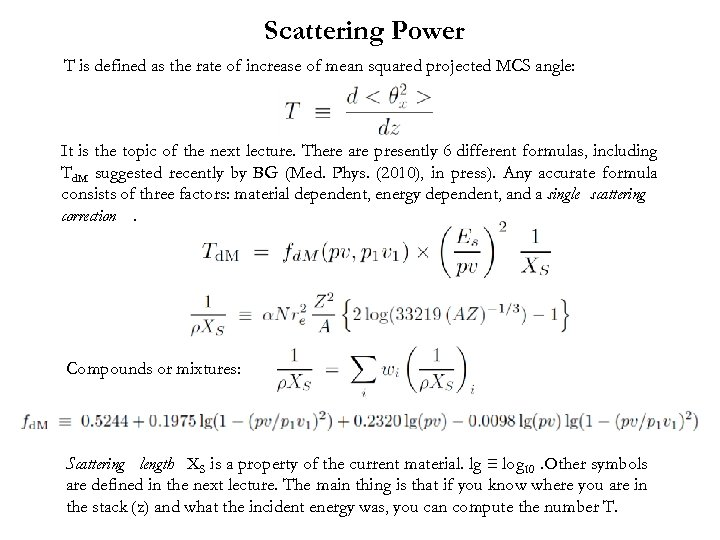Scattering Power T is defined as the rate of increase of mean squared projected MCS angle: It is the topic of the next lecture. There are presently 6 different formulas, including Td. M suggested recently by BG (Med. Phys. (2010), in press). Any accurate formula consists of three factors: material dependent, energy dependent, and a single scattering correction. Compounds or mixtures: Scattering length XS is a property of the current material. lg ≡ log 10. Other symbols are defined in the next lecture. The main thing is that if you know where you are in the stack (z) and what the incident energy was, you can compute the number T.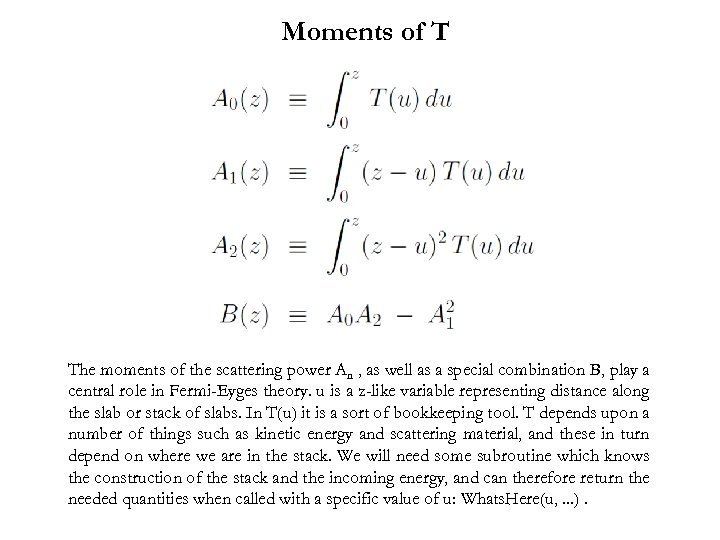Moments of T The moments of the scattering power An , as well as a special combination B, play a central role in Fermi-Eyges theory. u is a z-like variable representing distance along the slab or stack of slabs. In T(u) it is a sort of bookkeeping tool. T depends upon a number of things such as kinetic energy and scattering material, and these in turn depend on where we are in the stack. We will need some subroutine which knows the construction of the stack and the incoming energy, and can therefore return the needed quantities when called with a specific value of u: Whats. Here(u, . . . ).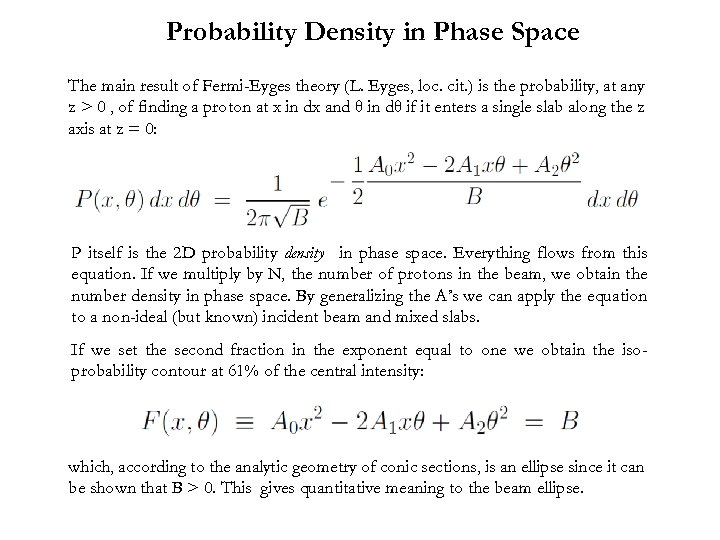Probability Density in Phase Space The main result of Fermi-Eyges theory (L. Eyges, loc. cit. ) is the probability, at any z > 0 , of finding a proton at x in dx and θ in dθ if it enters a single slab along the z axis at z = 0: P itself is the 2 D probability density in phase space. Everything flows from this equation. If we multiply by N, the number of protons in the beam, we obtain the number density in phase space. By generalizing the A’s we can apply the equation to a non-ideal (but known) incident beam and mixed slabs. If we set the second fraction in the exponent equal to one we obtain the isoprobability contour at 61% of the central intensity: which, according to the analytic geometry of conic sections, is an ellipse since it can be shown that B > 0. This gives quantitative meaning to the beam ellipse.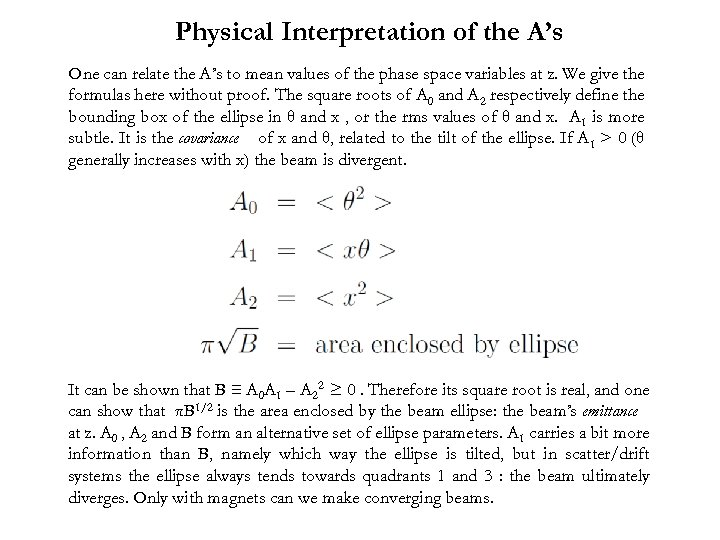Physical Interpretation of the A’s One can relate the A’s to mean values of the phase space variables at z. We give the formulas here without proof. The square roots of A 0 and A 2 respectively define the bounding box of the ellipse in θ and x , or the rms values of θ and x. A 1 is more subtle. It is the covariance of x and θ, related to the tilt of the ellipse. If A 1 > 0 (θ generally increases with x) the beam is divergent. It can be shown that B ≡ A 0 A 1 – A 22 ≥ 0. Therefore its square root is real, and one can show that πB 1/2 is the area enclosed by the beam ellipse: the beam’s emittance at z. A 0 , A 2 and B form an alternative set of ellipse parameters. A 1 carries a bit more information than B, namely which way the ellipse is tilted, but in scatter/drift systems the ellipse always tends towards quadrants 1 and 3 : the beam ultimately diverges. Only with magnets can we make converging beams.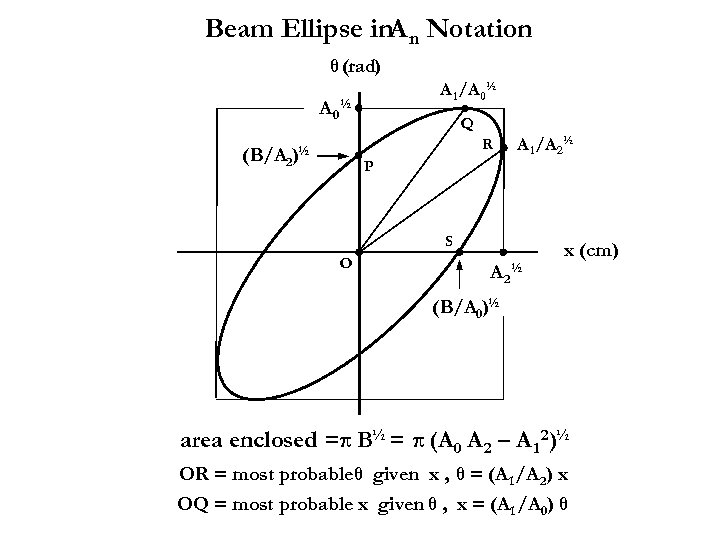Beam Ellipse in. A n Notation θ (rad) A 1 /A 0½ (B/A 2 Q R )½ P A 1 /A 2½ S O A 2 ½ x (cm) (B/A 0)½ area enclosed =π B½ = π (A 0 A 2 – A 1 2)½ OR = most probableθ given x , θ = (A 1 /A 2) x OQ = most probable x given θ , x = (A 1 /A 0) θ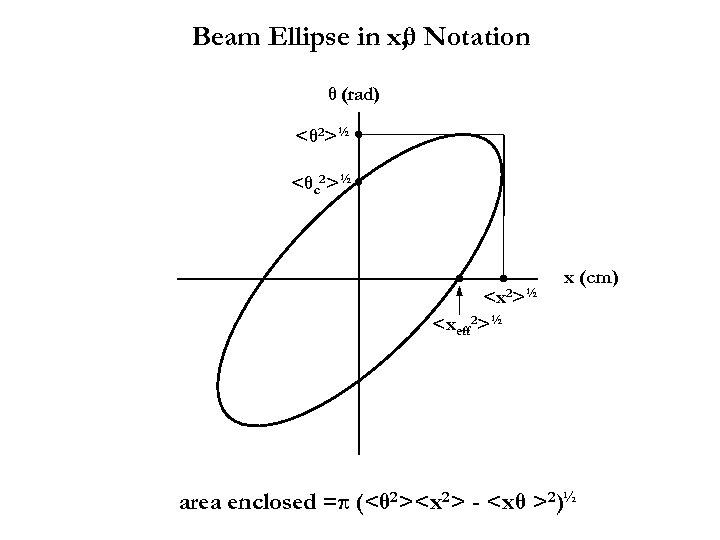Beam Ellipse in x, θ Notation θ (rad) <θ 2>½ <θc 2>½ ½ x (cm) area enclosed =π (<θ 2> - 2)½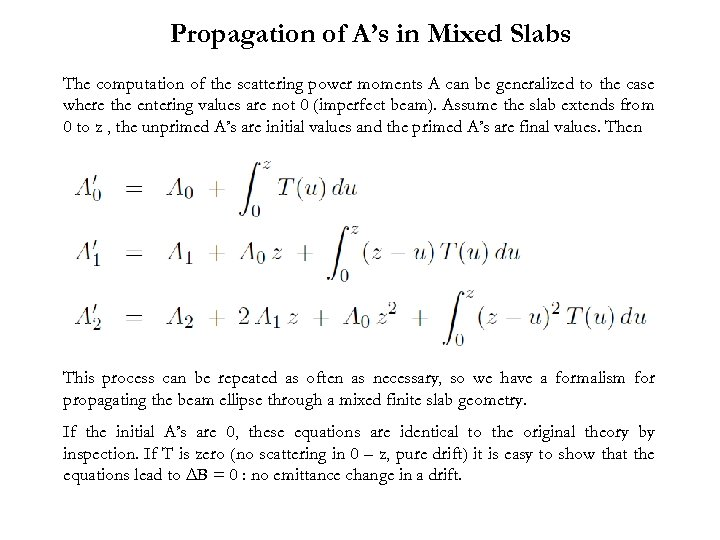Propagation of A’s in Mixed Slabs The computation of the scattering power moments A can be generalized to the case where the entering values are not 0 (imperfect beam). Assume the slab extends from 0 to z , the unprimed A’s are initial values and the primed A’s are final values. Then This process can be repeated as often as necessary, so we have a formalism for propagating the beam ellipse through a mixed finite slab geometry. If the initial A’s are 0, these equations are identical to the original theory by inspection. If T is zero (no scattering in 0 – z, pure drift) it is easy to show that the equations lead to ΔB = 0 : no emittance change in a drift.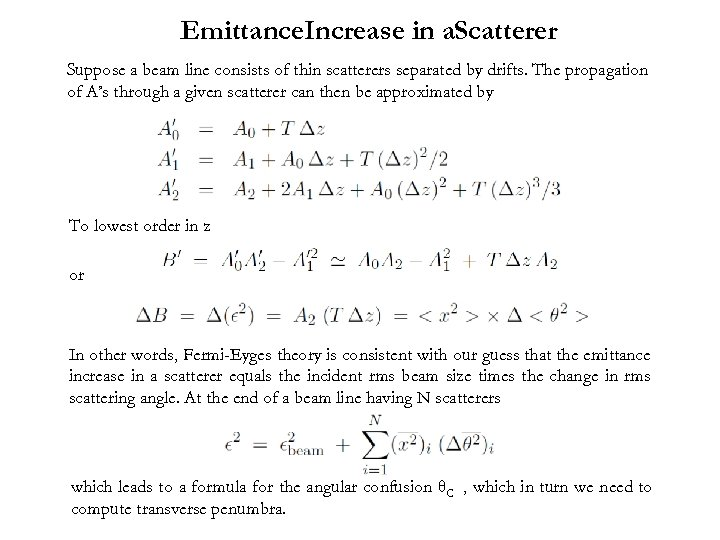Emittance. Increase in a. Scatterer Suppose a beam line consists of thin scatterers separated by drifts. The propagation of A’s through a given scatterer can then be approximated by To lowest order in z or In other words, Fermi-Eyges theory is consistent with our guess that the emittance increase in a scatterer equals the incident rms beam size times the change in rms scattering angle. At the end of a beam line having N scatterers which leads to a formula for the angular confusion θC , which in turn we need to compute transverse penumbra.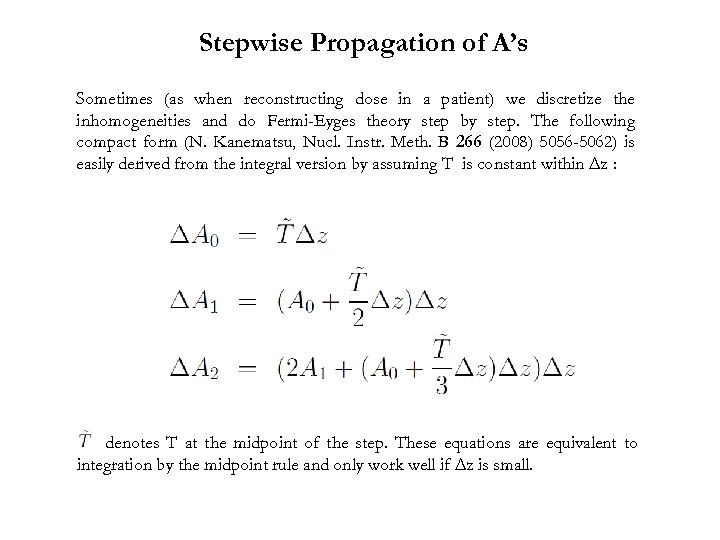Stepwise Propagation of A’s Sometimes (as when reconstructing dose in a patient) we discretize the inhomogeneities and do Fermi-Eyges theory step by step. The following compact form (N. Kanematsu, Nucl. Instr. Meth. B 266 (2008) 5056 -5062) is easily derived from the integral version by assuming T is constant within Δz : denotes T at the midpoint of the step. These equations are equivalent to integration by the midpoint rule and only work well if Δz is small.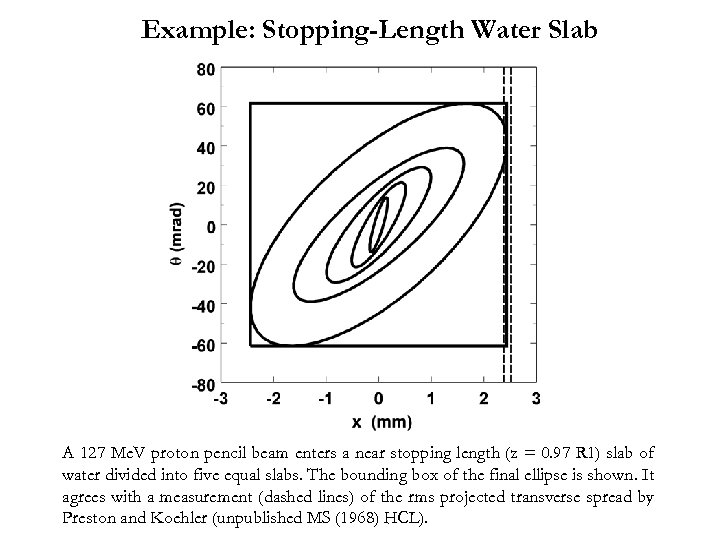Example: Stopping-Length Water Slab A 127 Me. V proton pencil beam enters a near stopping length (z = 0. 97 R 1) slab of water divided into five equal slabs. The bounding box of the final ellipse is shown. It agrees with a measurement (dashed lines) of the rms projected transverse spread by Preston and Koehler (unpublished MS (1968) HCL).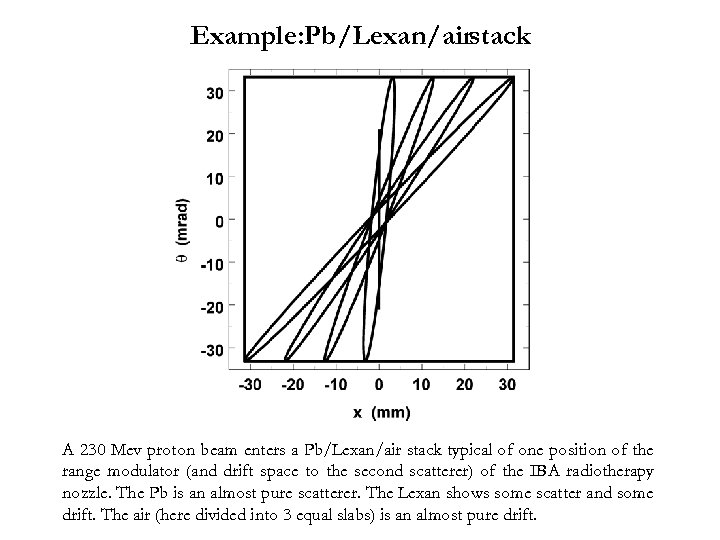Example: Pb/Lexan/airstack A 230 Mev proton beam enters a Pb/Lexan/air stack typical of one position of the range modulator (and drift space to the second scatterer) of the IBA radiotherapy nozzle. The Pb is an almost pure scatterer. The Lexan shows some scatter and some drift. The air (here divided into 3 equal slabs) is an almost pure drift.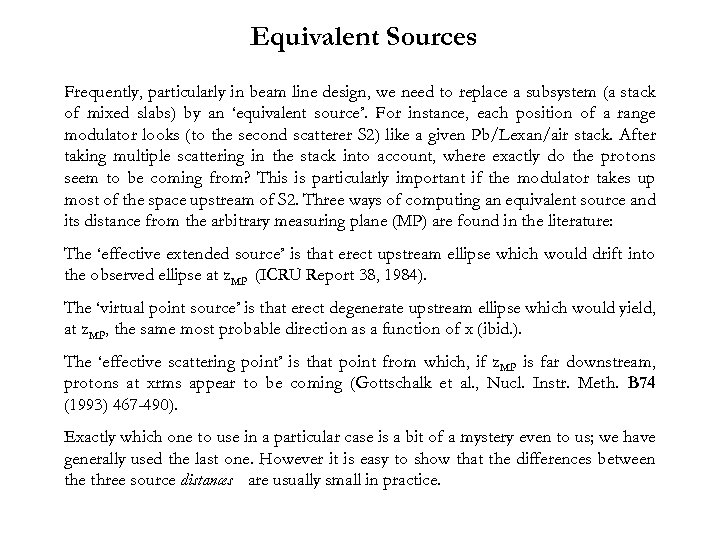Equivalent Sources Frequently, particularly in beam line design, we need to replace a subsystem (a stack of mixed slabs) by an ‘equivalent source’. For instance, each position of a range modulator looks (to the second scatterer S 2) like a given Pb/Lexan/air stack. After taking multiple scattering in the stack into account, where exactly do the protons seem to be coming from? This is particularly important if the modulator takes up most of the space upstream of S 2. Three ways of computing an equivalent source and its distance from the arbitrary measuring plane (MP) are found in the literature: The ‘effective extended source’ is that erect upstream ellipse which would drift into the observed ellipse at z. MP (ICRU Report 38, 1984). The ‘virtual point source’ is that erect degenerate upstream ellipse which would yield, at z. MP, the same most probable direction as a function of x (ibid. ). The ‘effective scattering point’ is that point from which, if z. MP is far downstream, protons at xrms appear to be coming (Gottschalk et al. , Nucl. Instr. Meth. B 74 (1993) 467 -490). Exactly which one to use in a particular case is a bit of a mystery even to us; we have generally used the last one. However it is easy to show that the differences between the three source distances are usually small in practice.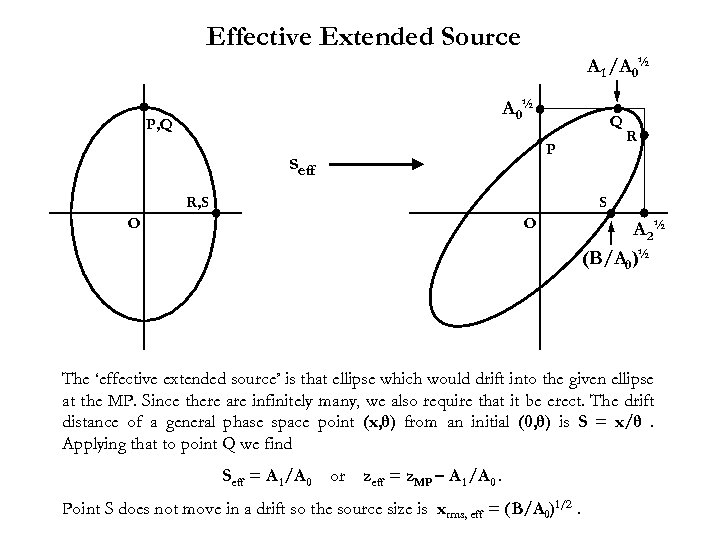Effective Extended Source A 1 /A 0½ P, Q Q P seff R, S R S O O A 2½ (B/A 0)½ The ‘effective extended source’ is that ellipse which would drift into the given ellipse at the MP. Since there are infinitely many, we also require that it be erect. The drift distance of a general phase space point (x, θ) from an initial (0, θ) is S = x/θ. Applying that to point Q we find Seff = A 1 /A 0 or zeff = z. MP – A 1 /A 0. Point S does not move in a drift so the source size is xrms, eff = (B/A 0)1/2.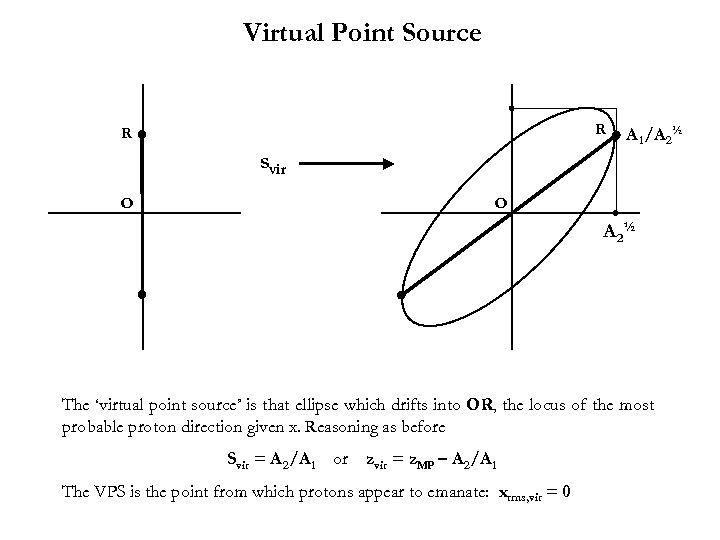Virtual Point Source R R A 1 /A 2½ svir O O A 2½ The ‘virtual point source’ is that ellipse which drifts into OR, the locus of the most probable proton direction given x. Reasoning as before Svir = A 2/A 1 or zvir = z. MP – A 2/A 1 The VPS is the point from which protons appear to emanate: xrms, vir = 0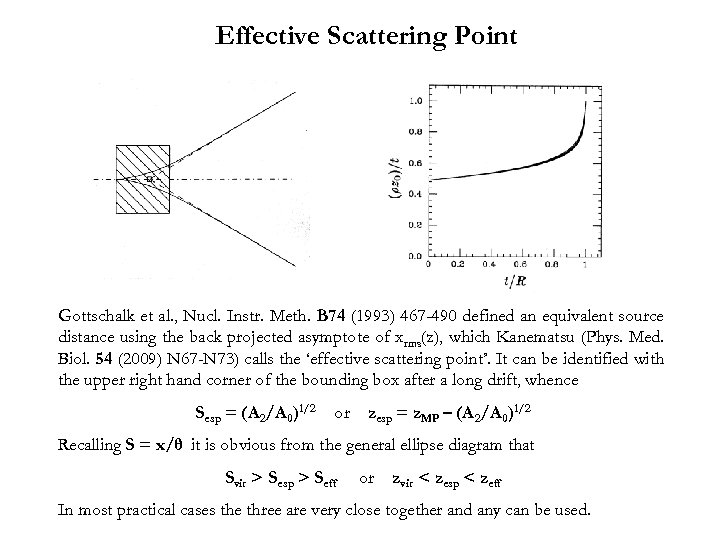Effective Scattering Point Gottschalk et al. , Nucl. Instr. Meth. B 74 (1993) 467 -490 defined an equivalent source distance using the back projected asymptote of xrms(z), which Kanematsu (Phys. Med. Biol. 54 (2009) N 67 -N 73) calls the ‘effective scattering point’. It can be identified with the upper right hand corner of the bounding box after a long drift, whence Sesp = (A 2/A 0)1/2 or zesp = z. MP – (A 2/A 0)1/2 Recalling S = x/θ it is obvious from the general ellipse diagram that Svir > Sesp > Seff or zvir < zesp < zeff In most practical cases the three are very close together and any can be used.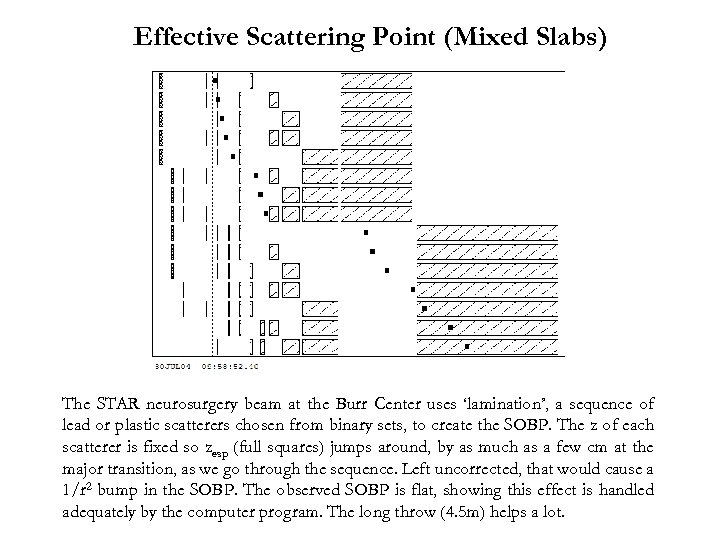Effective Scattering Point (Mixed Slabs) The STAR neurosurgery beam at the Burr Center uses ‘lamination’, a sequence of lead or plastic scatterers chosen from binary sets, to create the SOBP. The z of each scatterer is fixed so zesp (full squares) jumps around, by as much as a few cm at the major transition, as we go through the sequence. Left uncorrected, that would cause a 1/r 2 bump in the SOBP. The observed SOBP is flat, showing this effect is handled adequately by the computer program. The long throw (4. 5 m) helps a lot.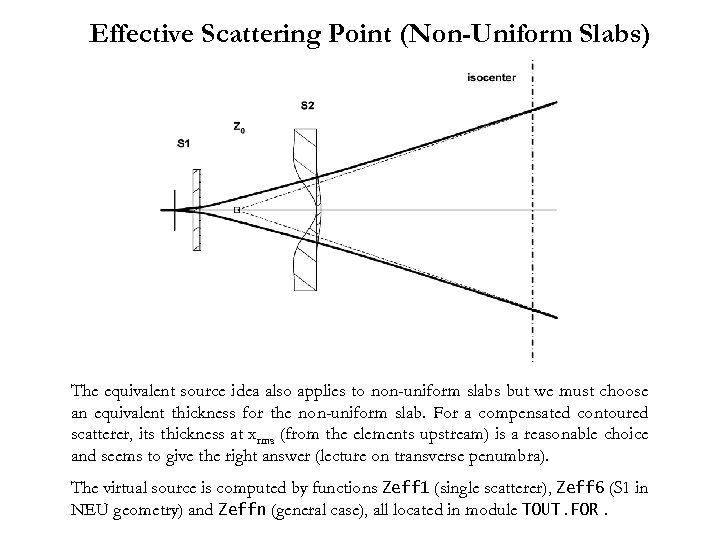Effective Scattering Point (Non-Uniform Slabs) The equivalent source idea also applies to non-uniform slabs but we must choose an equivalent thickness for the non-uniform slab. For a compensated contoured scatterer, its thickness at xrms (from the elements upstream) is a reasonable choice and seems to give the right answer (lecture on transverse penumbra). The virtual source is computed by functions Zeff 1 (single scatterer), Zeff 6 (S 1 in NEU geometry) and Zeffn (general case), all located in module TOUT. FOR.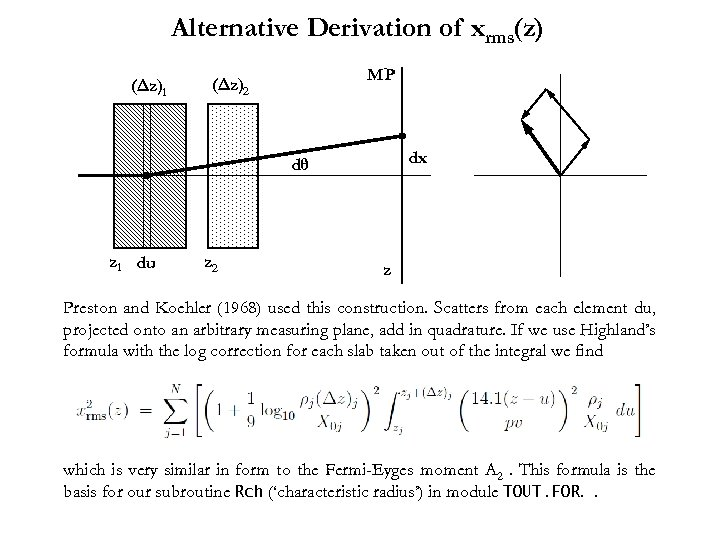Alternative Derivation of xrms(z) (Δz)1 MP (Δz)2 dx dθ z 1 du z 2 z Preston and Koehler (1968) used this construction. Scatters from each element du, projected onto an arbitrary measuring plane, add in quadrature. If we use Highland’s formula with the log correction for each slab taken out of the integral we find which is very similar in form to the Fermi-Eyges moment A 2. This formula is the basis for our subroutine Rch (‘characteristic radius’) in module TOUT. FOR. .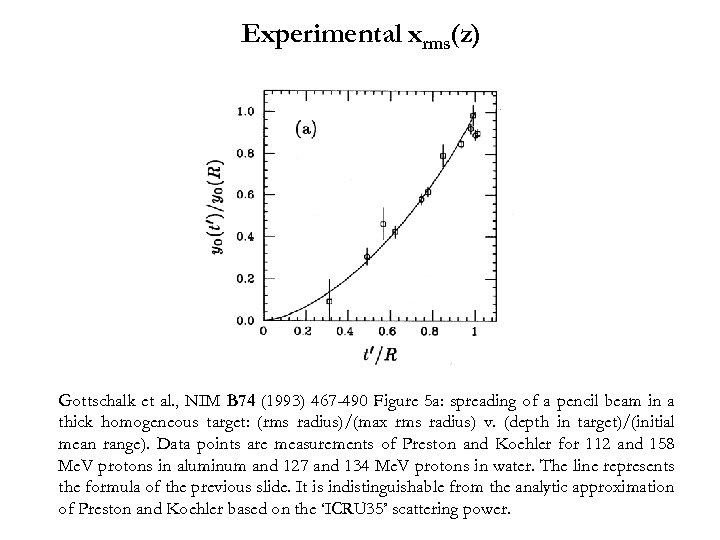Experimental xrms(z) Gottschalk et al. , NIM B 74 (1993) 467 -490 Figure 5 a: spreading of a pencil beam in a thick homogeneous target: (rms radius)/(max rms radius) v. (depth in target)/(initial mean range). Data points are measurements of Preston and Koehler for 112 and 158 Me. V protons in aluminum and 127 and 134 Me. V protons in water. The line represents the formula of the previous slide. It is indistinguishable from the analytic approximation of Preston and Koehler based on the ‘ICRU 35’ scattering power.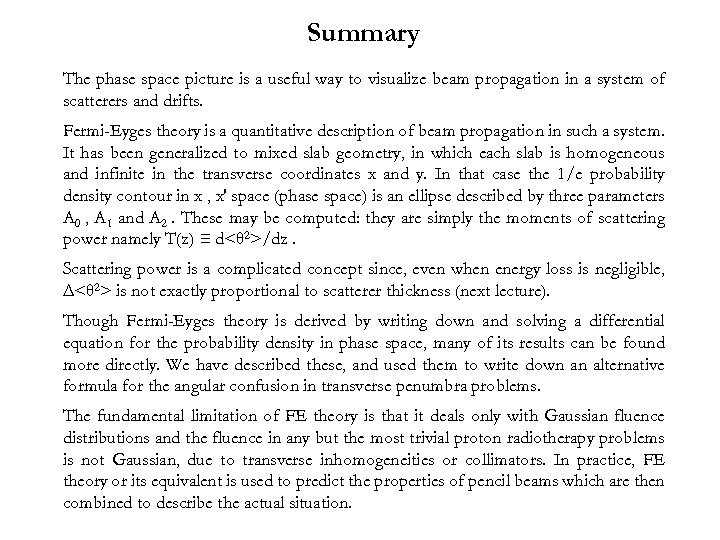Summary The phase space picture is a useful way to visualize beam propagation in a system of scatterers and drifts. Fermi-Eyges theory is a quantitative description of beam propagation in such a system. It has been generalized to mixed slab geometry, in which each slab is homogeneous and infinite in the transverse coordinates x and y. In that case the 1/e probability density contour in x , x′ space (phase space) is an ellipse described by three parameters A 0 , A 1 and A 2. These may be computed: they are simply the moments of scattering power namely T(z) ≡ d<θ 2>/dz. Scattering power is a complicated concept since, even when energy loss is negligible, Δ<θ 2> is not exactly proportional to scatterer thickness (next lecture). Though Fermi-Eyges theory is derived by writing down and solving a differential equation for the probability density in phase space, many of its results can be found more directly. We have described these, and used them to write down an alternative formula for the angular confusion in transverse penumbra problems. The fundamental limitation of FE theory is that it deals only with Gaussian fluence distributions and the fluence in any but the most trivial proton radiotherapy problems is not Gaussian, due to transverse inhomogeneities or collimators. In practice, FE theory or its equivalent is used to predict the properties of pencil beams which are then combined to describe the actual situation.